# Feature Encoding Techniques – Machine Learning

As we all know that better encoding leads to a better model and most algorithms cannot handle the categorical variables unless they are converted into a numerical value.

Categorical features are generally divided into 3 types:

A. Binary: Either/or
Examples:

• Yes, No
• True, False

B. Ordinal: Specific ordered Groups.
Examples:

• low, medium, high
• cold, hot, lava Hot

C. Nominal: Unordered Groups. Examples

• cat, dog, tiger
• pizza, burger, coke

Example:

## Python3

 `# data preprocessing` `import` `pandas as pd  ` `# for linear calculations` `import` `numpy as np    ` `# Plotting Graphs` `import` `seaborn as sns ` `df ``=` `pd.read_csv(``"Encoding Data.csv"``)` `# displaying top 10 results` `df.head(``10``)   `

Output: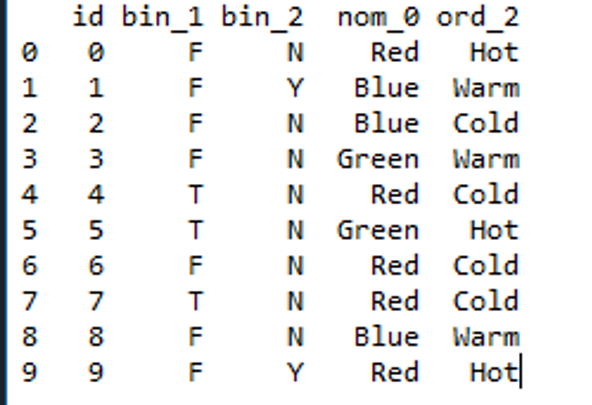Dataset

Let’s examine the columns of the dataset with different types of encoding techniques.

Code: Mapping binary features present in the dataset.

## Python3

 `# you can always use simple mapping on binary features.` `df[``'bin_1'``] ``=` `df[``'bin_1'``].``apply``(` `    ``lambda` `x: ``1` `if` `x ``=``=` `'T'` `else` `(``0` `if` `x ``=``=` `'F'` `else` `None``))` `df[``'bin_2'``] ``=` `df[``'bin_2'``].``apply``(` `    ``lambda` `x: ``1` `if` `x ``=``=` `'Y'` `else` `(``0` `if` `x ``=``=` `'N'` `else` `None``))` `sns.countplot(df[``'bin_1'``])` `sns.countplot(df[``'bin_2'``])`

Output: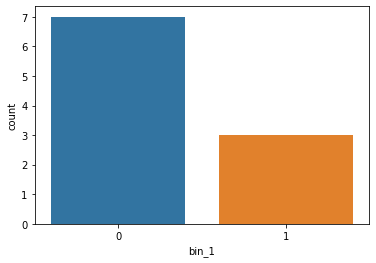Bin_1 after applying mapping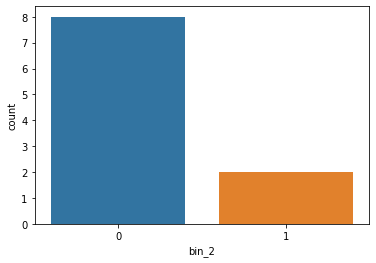bin_2 after applying mapping

Label Encoding: Label encoding algorithm is quite simple and it considers an order for encoding, Hence can be used for encoding ordinal data.

Code:

## Python3

 `# labelEncoder present in scikitlearn library` `from` `sklearn.preprocessing ``import` `LabelEncoder  ` `le ``=` `LabelEncoder()` `df[``'ord_2'``] ``=` `le.fit_transform(df[``'ord_2'``])` `sns.``set``(style ``=``"darkgrid"``)` `sns.countplot(df[``'ord_2'``])`

Output: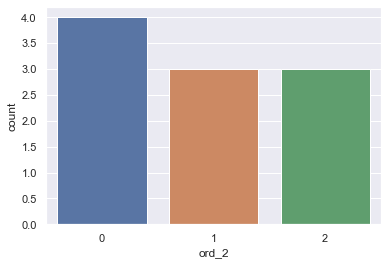Plot of ord_2 after label encoding

One-Hot Encoding: To overcome the Disadvantage of Label Encoding as it considers some hierarchy in the columns which can be misleading to nominal features present in the data. we can use the One-Hot Encoding strategy.
One-hot encoding is processed in 2 steps:

1. Splitting of categories into different columns.
2. Put ‘0 for others and ‘1’ as an indicator for the appropriate column.

Code: One-Hot encoding with Sklearn library

## Python3

 `from` `sklearn.preprocessing ``import` `OneHotEncoder` `enc ``=` `OneHotEncoder()` `# transforming the column after fitting` `enc ``=` `enc.fit_transform(df[[``'nom_0'``]]).toarray()` `# converting arrays to a dataframe` `encoded_colm ``=` `pd.DataFrame(enc)` `# concatenating dataframes` `df ``=` `pd.concat([df, encoded_colm], axis``=``1``)` `# removing the encoded column.` `df ``=` `df.drop([``'nom_0'``], axis``=``1``)` `df.head(``10``)`

Output: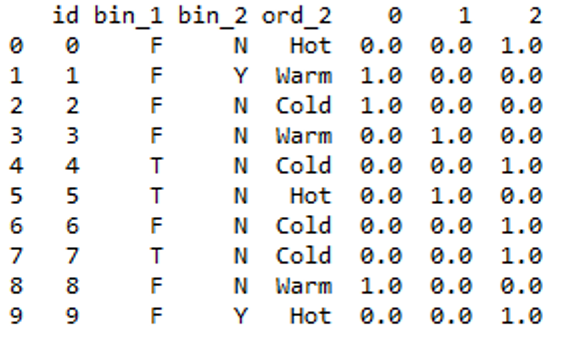Output

Code: One-Hot encoding with pandas

## Python3

 `df ``=` `pd.get_dummies(df, prefix``=``[``'nom_0'``], columns``=``[``'nom_0'``])` `df.head(``10``)`

Output: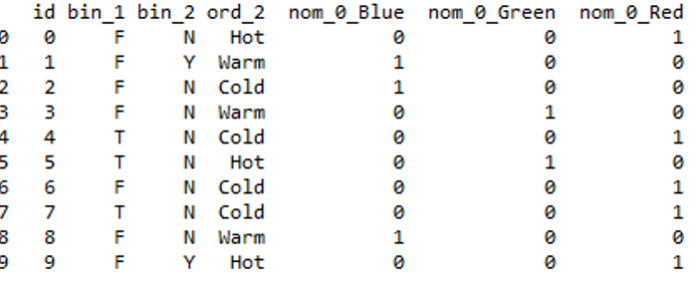output

This method is preferable since it gives good labels.

Note: One-hot encoding approach eliminates the order but it causes the number of columns to expand vastly. So for columns with more unique values try using other techniques.

Frequency Encoding: We can also encode considering the frequency distribution. This method can be effective at times for nominal features.

Code:

## Python3

 `# grouping by frequency` `fq ``=` `df.groupby(``'nom_0'``).size()``/``len``(df)` `# mapping values to dataframe` `df.loc[:, ``"{}_freq_encode"``.``format``(``'nom_0'``)] ``=` `df[``'nom_0'``].``map``(fq)` `# drop original column.` `df ``=` `df.drop([``'nom_0'``], axis``=``1``)` `fq.plot.bar(stacked``=``True``)` `df.head(``10``)`

Output: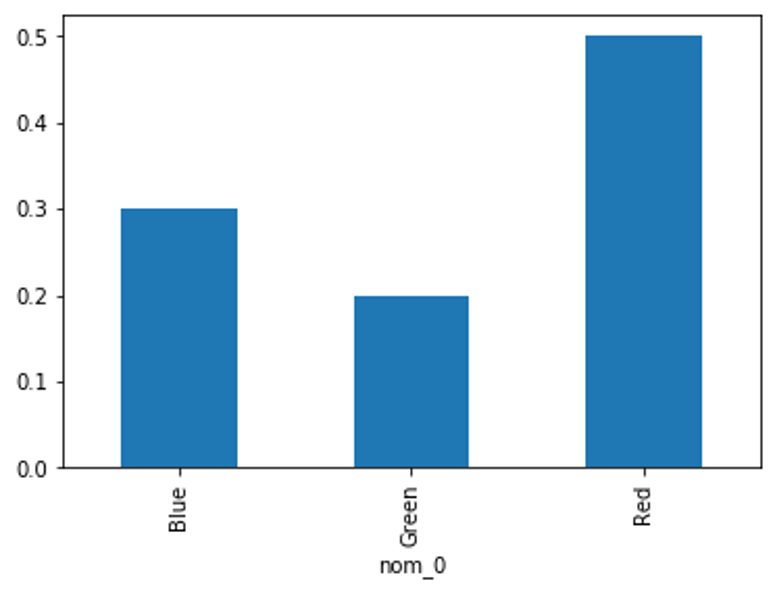Frequency distribution (fq)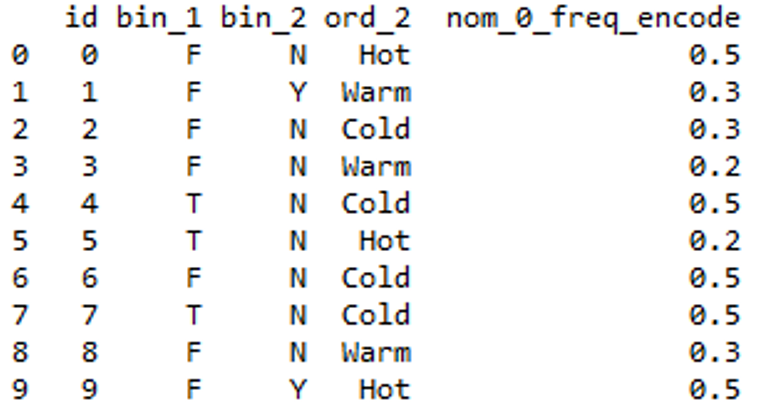Output

Ordinal Encoding: We can use Ordinal Encoding provided in Scikit learn class to encode Ordinal features. It ensures that ordinal nature of the variables is sustained.

Code: Using Scikit learn.

## Python3

 `from` `sklearn.preprocessing ``import` `OrdinalEncoder` `ord1 ``=` `OrdinalEncoder()` `# fitting encoder` `ord1.fit([df[``'ord_2'``]])` `# transforming the column after fitting` `df[``"ord_2"``] ``=` `ord1.transform(df[[``"ord_2"``]])` `df.head(``10``)`

Output: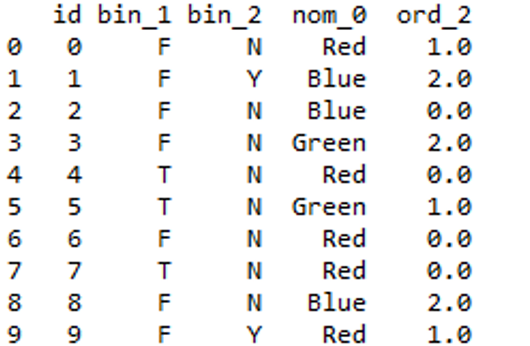Output

One issue with this representation (Ordinal Encoding) is that the ML algorithm would assume that the two nearby values are closer than the distinct ones.

Example of the above Problem:

## Python3

 `from` `sklearn.preprocessing ``import` `OrdinalEncoder` `x``=``[[``"red"``,``"green"``],[``"yellow"``,``"red"``]]` `ord``=``OrdinalEncoder()` `output``=``ord``.fit_transform(x)` `print``(output)`

Output: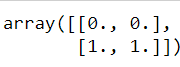It’s looking for the most nearby ones. It assumes that “red” and “green” belong to the same category.

Code: Manually assigning ranking by using a dictionary

## Python3

 `# creating a dictionary` `temp_dict ``=` `{``'Cold'``: ``1``, ``'Warm'``: ``2``, ``'Hot'``: ``3``}` `# mapping values in column from dictionary` `df[``'Ord_2_encod'``] ``=` `df.ord_2.``map``(temp_dict)` `df ``=` `df.drop([``'ord_2'``], axis``=``1``)` ` Output: < ``/``strong >`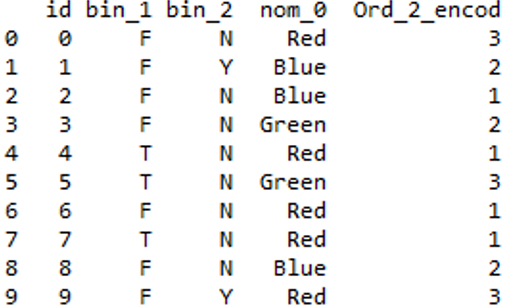Output

Binary Encoding: Initially, categories are encoded as Integer and then converted into binary code, then the digits from that binary string are placed into separate columns.

`for eg: for 7 : 1 1 1 `

This method is quite preferable when there is more categories. Imagine if you have 100 different categories. One hot encoding will create 100 different columns, But binary encoding only need 7 columns.

Code:

## Python3

 `from` `category_encoders ``import` `BinaryEncoder ` `encoder ``=` `BinaryEncoder(cols ``=``[``'ord_2'``]) ` `# transforming the column after fitting` `newdata ``=` `encoder.fit_transform(df[``'ord_2'``])` `# concatenating dataframe` `df ``=` `pd.concat([df, newdata], axis ``=` `1``) ` `# dropping old column ` `df ``=` `df.drop([``'ord_2'``], axis ``=` `1``)` `df.head(``10``)`

Output: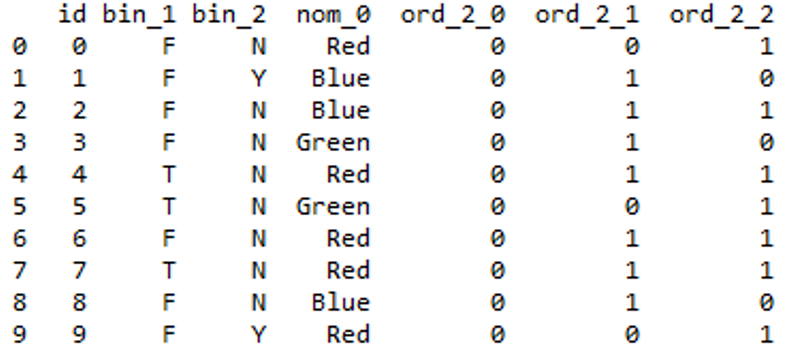Output

HashEncoding: Hashing is the process of converting of a string of characters into a unique hash value with applying a hash function. This process is quite useful as it can deal with a higher number of categorical data and its low memory usage.
Article regarding hashing

Code:

## Python3

 `from` `sklearn.feature_extraction ``import` `FeatureHasher` `# n_features contains the number of bits you want in your hash value.` `h ``=` `FeatureHasher(n_features ``=` `3``, input_type ``=``'string'``) ` `# transforming the column after fitting` `hashed_Feature ``=` `h.fit_transform(df[``'nom_0'``])` `hashed_Feature ``=` `hashed_Feature.toarray()` `df ``=` `pd.concat([df, pd.DataFrame(hashed_Feature)], axis ``=` `1``)` `df.head(``10``)`

Output: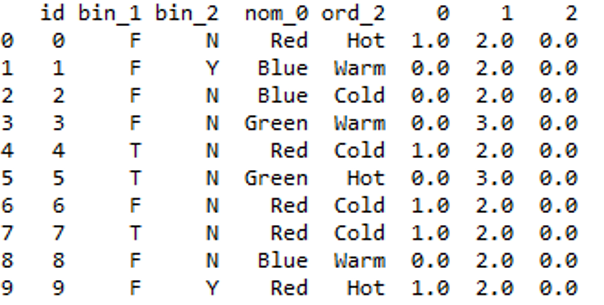Output

You can further drop the converted feature from your Dataframe.

Mean/Target Encoding: Target encoding is good because it picks up values that can explain the target. It is used by most kagglers in their competitions. The basic idea is to replace a categorical value with the mean of the target variable.

Code:

## Python3

 `# inserting Target column in the dataset since it needs a target` `df.insert(``5``, ``"Target"``, [``0``, ``1``, ``1``, ``0``, ``0``, ``1``, ``0``, ``0``, ``0``, ``1``], ``True``) ` `# importing TargetEncoder` `from` `category_encoders ``import` `TargetEncoder` `Targetenc ``=` `TargetEncoder()` `# transforming the column after fitting` `values ``=` `Targetenc.fit_transform(X ``=` `df.nom_0, y ``=` `df.Target)` `# concatenating values with dataframe` `df ``=` `pd.concat([df, values], axis ``=` `1``)` `df.head(``10``)`

You can further drop the converted feature from your Dataframe.

Output: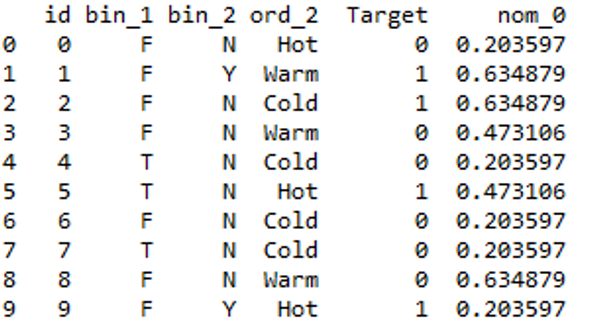output

Whether you're preparing for your first job interview or aiming to upskill in this ever-evolving tech landscape, GeeksforGeeks Courses are your key to success. We provide top-quality content at affordable prices, all geared towards accelerating your growth in a time-bound manner. Join the millions we've already empowered, and we're here to do the same for you. Don't miss out - check it out now!